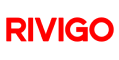New update is available. Click here to update.
Topics

# Median in a stream

Hard0/120
Average time to solve is 50m+21 more companies

## Problem statement

Median is the middle value in an ordered integer list. If the size of the list is even there is no middle value. So the median is the floor of the average of the two middle values.

For example :
``````[2,3,4] - median is 3.
[2,3] - median is floor((2+3)/2) = 2.
``````

Detailed explanation ( Input/output format, Notes, Images )
Constraints :
``````1 <= T <= 10
1 <= N <= 10^4
0 <= data <= 10^8
Where T is the number of test cases, N is the number of elements in the data stream.

Time Limit : 1 sec
``````
Sample Input 1:
``````3
3
1 2 3
2
9 9
1
4
``````
Sample Output 1:
``````1 1 2
9 9
4
``````
Explanation for Sample Input 1:
``````For test case 1
After reading first element of stream , Median of  is 1
After reading second element of stream, Median of [1, 2]  = floor((1+2)/2)  = 1
After reading third element of stream, Median of [1,2,3] = 2
So the output will be 1 1 2.
``````
Sample Input 2:
``````2
3
5 3 8
2
3 8
``````
Sample Output 2:
``````5 4 5
3 5
``````
Explanation for Sample Input 2:
``````For test case 1
After reading first element of stream, Median of  is 5
After reading second element of stream, Median of [5, 3]  = floor((5+3)/2)  = 4
After reading third element of stream, Median of [5,3,8] = 5 , it is the middle value of the sorted array
So the output will be 5 4 5.
``````Console